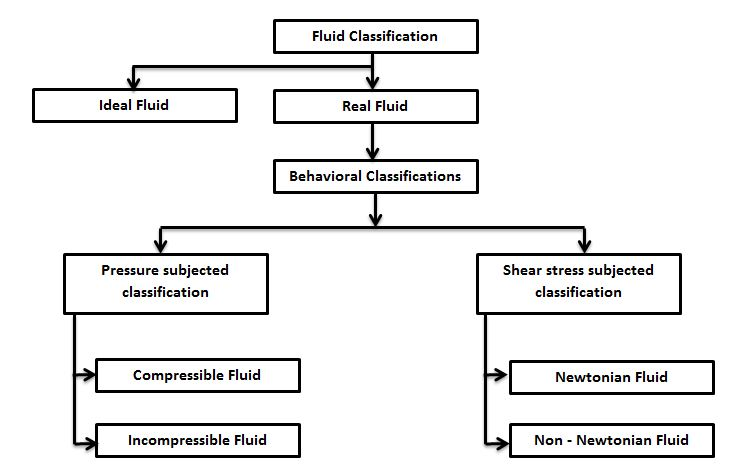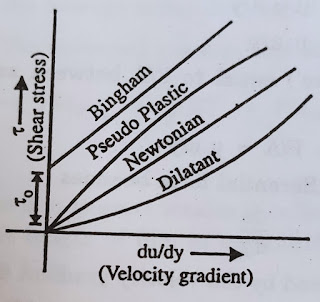# Fluid mechanics - Density, Specific Gravity, Viscosity, Vapour pressure etc

## Fluid mechanics - Density, Specific Gravity, Viscosity, Vapour pressure etc

Fluid mechanics - Density, Specific Gravity, Viscosity, Vapour pressure etc contains detailed study for industrial persons in easy terms

### Blog Contents -

• Fluid mechanics [Fluid properties]
• Classification of fluids
• Density of fluid.
• Weight density
• Specific volume
• Specific Gravity of fluid.
• Viscosity of fluid.
• Vapour pressure of fluid.

### Interview Questions from this blog

1. What is definition of fluid?
2. Classifications of fluid
3. What are Newtonian fluids and Non Newtonian fluids?
4. Why density is depend on Temperature & Pressure?
5. What is classification of Non Newtonian fluid?
6. What is relation between mass density & mass density?
7. What is relation between Vapour Pressure & Temperature?
8. How vapour change with molecular forces?

### Fluid definitions

Fluid is a substance which under goes continuous deformation (flows) when subjected to external force or gravity.

Other definitions
1. Fluid is a substance which one’s particles easily move and change their respective position with other particles when substance is free or under pressure or under gravity
2. Fluid is capable to flow when allowed to flow freely. or Fluid is a substance tends to conform to the containers outline or free to flow
3. Fluid has no definite shape but when it fill in any Container it follow container's shape called Fluid

### Classification of fluid

Fluid classification is as per belowClassification of fluid

#### Definitions of Fluids of each type:

Ideal Fluid -
• Ideal fluid is a fluid which does not offer any resistance in flow / deformation / shape change.
• Fluid doesn't has viscosity
• It is friction-less & In compressible.
• Ideal fluid is not exists It is imaginary fluid.
Real Fluid
• Fluid found in nature and reality called real fluid.
• Real fluid offers resistance when it is in motion.
Compressible fluid
• Fluids which's density depends on Temperature & Pressure called Compressible fluid.
• Fluid of which the density is sensible to change in temperature or pressure called Compressible fluid
• Generally gases are compressible fluids.
Incompressible fluids
• If density of fluid is not affected by change in temperature or pressure applied in temperature that fluid is called Incompressible fluid.
• Generally liquids are incompressible
Newtonian Fluid
• Fluids which follows Newton's law of viscosity are called Newtonian fluids.
• Newton's law of viscosity :  The fluids for which the ratio of the shear stress to the rate of shear is constant
Non Newtonian fluids
• Fluids which are not following newton's law of viscosity calling Non Newtonian fluid

### Density of fluid [Mass density]

• Density ⍴ or mass density of fluid is ratio of Mass of fluid m per unit Volume V
• SI unit of Density is Kg/m3
• Density of water at 4℃ [277K] is 1000 kg/m3
Density ⍴ = Mass (m) / Unit Volume (V)
• Density is dependent on Temperature and Pressure.
• As per Ideal Gas Law
PV = nRT
• When Temperature is increases Volume of fluids increases.
• When Pressure is Increases Volume of fluid decreases.
• Generally Density of fluid is not much change in slight change in Temperature.
• Gas density is change higher than liquid in raise temperature.

Example of Density -
Copper cube sink in water, but in mercury it float.

#### Case 1 : Copper Cube in Mercury

Why? -
Density of Mercury : 13600 kg/m3
Density of Copper : 9000 kg/m3

Copper is light in comparison.
This is the reason Copper cube if floating

#### Case 1 : Copper Cube in Water

Why? -
Density of Water : 999 kg/m3
Density of Copper : 9000 kg/m3

Water is light in comparison.
This is the reason Copper cube if floating

### Weight density

• Weight of fluid per unit volume.
• Weight density of Water at 4°C : 9810 N/m3
• Relation between Mass density & Weight density
w = ⍴ g
• g = gravitational force 9.81 m/s2

### Specific Volume

• It is volume in unit mass
• unit : m3/kg

### Specific Gravity [ Sp.Gr., Relative Density]

Its ratio of Density of fluid to Density of standard fluid.

Standard fluids
Liquid - Water at 4℃, 1 Atmospheric pressure.
Air - Air at 20℃, 1 Atmospheric

Unit : Unit less [You can consider kg/m3]
Unit Calculation -

### Viscosity

Fluid is a substance able to flow but there is resistance in flow is called Viscosity
• Honey has more viscosity so it is not flow freely.
• Water has less viscosity so it flows more freely.
• It is not depend on Pressure.
• Viscosity decrease when temperature increase. [ When temperature rises molecular vibration also increases which makes fluid free and reduce it's viscosity].
• Viscosity governed by Cohesive forces[Attractive forces].

#### Viscosity calculation

Force is proportional to velocity and area and inversely proportional to Distance
F ∝ u A / Y
F = Force required to move fluid from two parallel layers 1 & 2.

F = μ u A / Y

μ = Viscosity of fluid
Coefficient of Viscosity, Dynamic Viscosity

F / A = μ u / Y
𝛕 = F / A
𝛕 = μ * u / Y
We need velocity gradient to measure viscosity.
Velocity gradient is velocity of fluid with respect to distance traveled.
μ  = 𝛕 / (du/dY)
This is Newton's law of viscosity
• Viscosity μ is Shear stress required to produce unit flow [Shear deformation].
• Unit : N.s/m^2 [SI Unit]
• Kinetic Viscosity of fluid is ration of viscosity of fluid to density. 𝝂 = μ/⍴

### Newtonian & Non Newtonian fluid

#### Newtonian fluid definitions

1. Shear stress to Rate of shear Ratio of  is constant
2. Fluids which has velocity gradient equal to Shear stress applied.
3. Fluid which has 45° angle graph in Shear stress  vs Velocity gradient du/dy
4. Examples - All gases, Air; Liquids - Kerosene, alcohol, glycerin.#### Non Newtonian fluid definitions

1. Fluid for which  Share stress vs Velocity gradient is not constant
2. Fluids not follow Newton's law of Viscosity.
Examples - There are three types of Newtonian fluids
1. Bingham fluids
2. Pseudo plastic
3. Dilatent fluid
1. Bingham fluids
• When Shear stress is small it resist to flow.
• When Shell stress is larger it follow linear shear stress vs flow called Bingham fluids of Plastics.
• Examples - Toothpaste, Jellies, Paints, Slurries.
• 𝜏 = 𝜏0; du/dy=0; 𝜏>𝜏0 𝜏=𝜏0 + η du/dy. 𝜏0 = yield stress, η = rigidity coefficient
2. Pseudo plastic or fluids [shear rate thinning fluid]
• Viscosity decrease with increase in velocity.
• Examples - Blood, High molecular weight solution, paper pulp, rubber latex.
3. Dilatent fluids [shear rate ticking fluid]
• Viscosity increases when velocity increases.
• Examples - Suspensions of starch, pulp in water, emulsions.
Experimental curve of Shear stress vs Velocity given by Ostwald-de-waele equation

𝜏 = k (du/dy)n

Where k & n are arbitrary

n = 1 k = μ : Newtonian fluid
n < 1 : Pseudo plastic
n > 1 : Dilatant fluids

### Vapour Pressure

Pressure formed by vapour of liquid over the surface of liquid in closed container which is in thermodynamic equilibrium state at a given temperature.
• Pure water's Vapour pressure is 1 atmosphere at 100℃
• All liquids have tendency to vaporize.
• Molecules of liquid escaping through free surface of liquid to space.
• Vapour accumulation develop pressure on liquid surface and towards container vessel.
The density of gas is related to absolute pressure and absolute temperature
PMavg = ρRT
Examples:

#### Vapour pressure vs Temperature

• Vapour pressure increase with increase in temperature
• Water Vapour pressure Vs Temperature Graph

• Vapour pressure is not affected by surface area.
• Water vapour pressure is same on small container & large vessels.
• Vapour pressure is depends on dipole-dipole forces & London dispersion forces.

Wikipedia -
Fluid
SI Unit
Density
Specific Gravity
Viscosity
Vapour Pressure

Fluid

Viscosity

Vapour Pressure